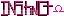-=+=- -=+=- -=+=- -=+=- -=+=- -=+=- -=+=- -=+=- -=+=- -=+=- -=+=- -=+=- -=+=- -=+=- -=+=- -=+=- -=+=- -=+=- -=+=- -=+=- -=+=- -=+=- -=+=- -=+=- -=+=- -=+=- -=+=- -=+=- -=+=- -=+=- (c) WidthPadding Industries 1987 0|433|0 -=+=- -=+=- -=+=- -=+=- -=+=- -=+=- -=+=- -=+=- -=+=- -=+=- -=+=- -=+=- -=+=- -=+=- -=+=- -=+=- -=+=- -=+=- -=+=- -=+=- -=+=- -=+=- -=+=- -=+=- -=+=- -=+=- -=+=- -=+=- -=+=- -=+=-
SoCoder -> Link Home -> Videos

spinalCreated : 26 May 2008

### Hornophone!

Monday, 26 May 2008, 16:16
HoboBenWOW!

That was really quite epic.
Monday, 26 May 2008, 19:46
steve_ancellI was on a bit of a downer until I watched that, that's cheered my up. COOL.
Saturday, 31 May 2008, 08:31
Scherererer0_0 wow. I wonder how much time it took him to set all that up.Monday, 09 June 2008, 02:15The A-Team bit was special